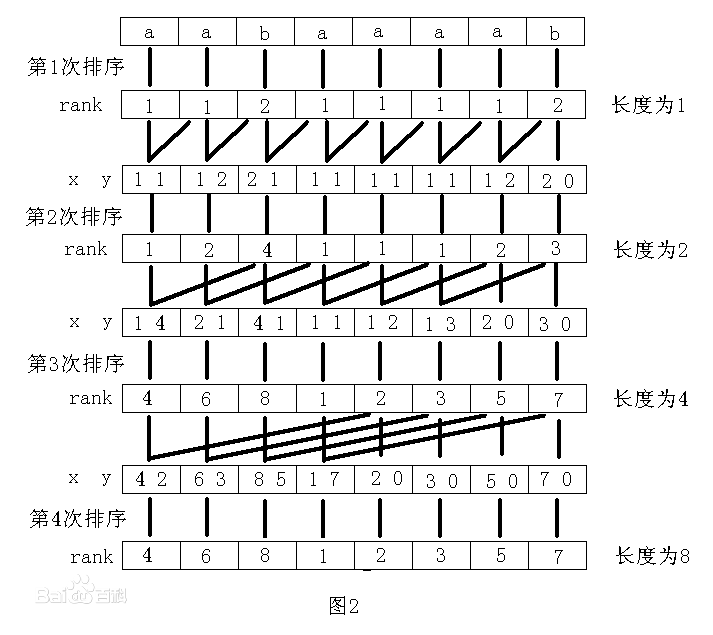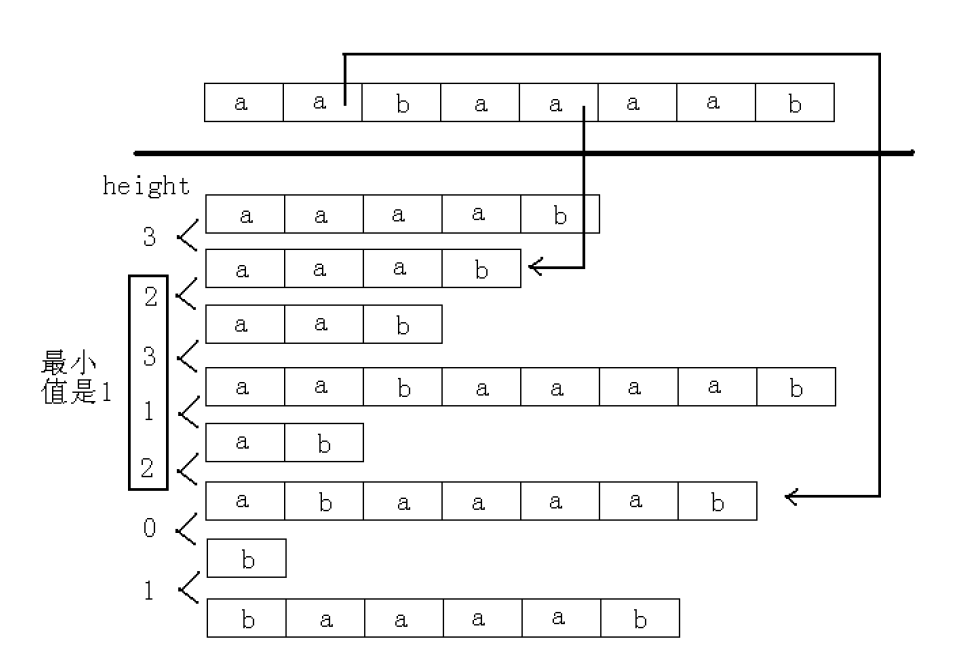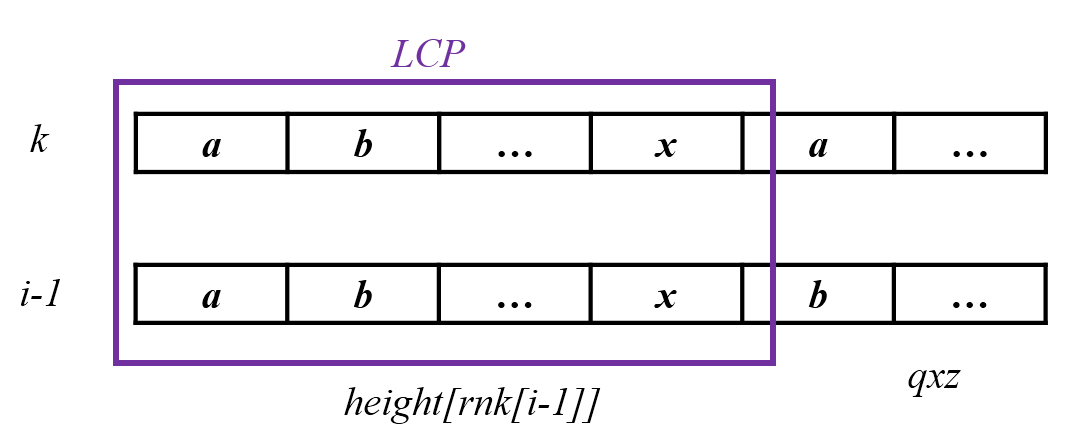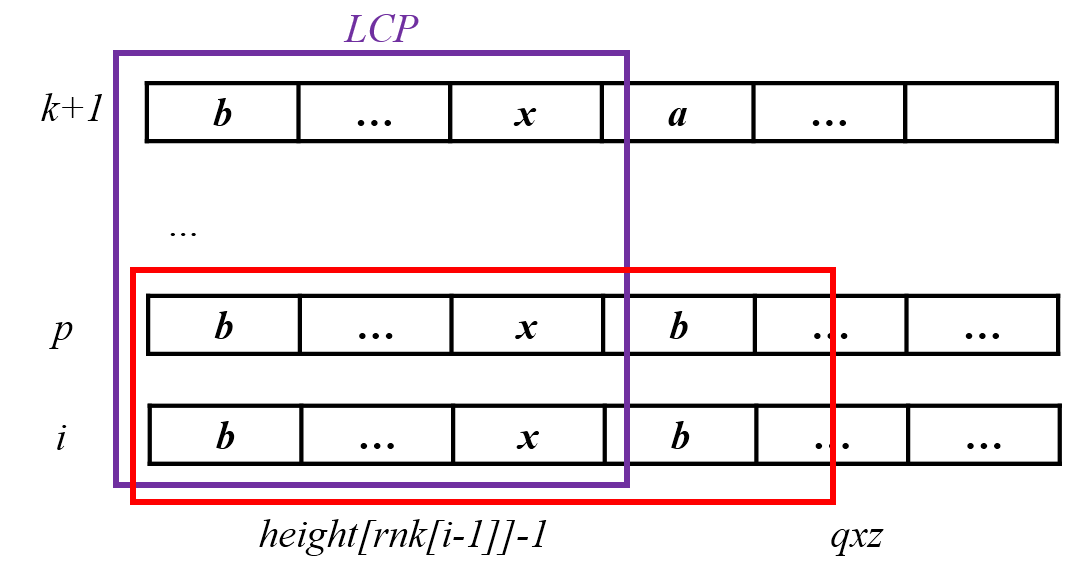# 「后缀数组」学习笔记

$Manber$ & $Mayer$倍增法，思想很好理解。由于各个后缀之间不像普通的数列，它们是有内部联系的：即后缀关系。因此我们想到每次对所有后缀的前$2^k$位排序。而后在对$2^{k+1}$排序的时候充分利用排$2^k$时的信息来完成。由于已对所有$2^k$进行了排序，也就知道了所有$2^k$的相对关系。这样在排$2^{k+1}$时相当于是在排二元组，即问题转化为了二元组的快速排序问题。而这能够利用$O(n)$的基数排序实现。因此后缀数组的构造复杂度是$O(n \log n)$inline void get_SA(int m){
int p;
for(int i = 1; i <= m; ++i) c[i] = 0;
for(int i = 1; i <= n; ++i) ++c[x[i] = s[i]];
for(int i = 1; i <= m; ++i) c[i] += c[i-1];
for(int i = n; i >= 1; --i) sa[c[x[i]]--] = i;
for(int k = 1; k <= n; k += k){
p = 0;
for(int i = n-k+1; i <= n; ++i) y[++p] = i;
for(int i = 1; i <= n; ++i) if(sa[i] > k) y[++p] = sa[i]-k;
for(int i = 1; i <= m; ++i) c[i] = 0;
for(int i = 1; i <= n; ++i) ++c[x[i]];
for(int i = 1; i <= m; ++i) c[i] += c[i-1];
for(int i = n; i >= 1; --i) sa[c[x[y[i]]]--] = y[i], y[i] = 0;
swap(x,y);
x[sa] = 1, p = 1;
for(int i = 2; i <= n; ++i) x[sa[i]] = (y[sa[i]]==y[sa[i-1]] && y[sa[i]+k]==y[sa[i-1]+k]) ? p : ++p;
if(p >= n) break;
m = p;
}
}POJ 2774 Long Long Message

luogu 3763 DNA

luogu 2852 Milk Patterns

luogu 3975 弦论

luogu 4051 字符加密

luogu 3966 单词

luogu 4248 差异

luogu 3181 找相同字符

</font>

posted @ 2019-06-13 12:16  DennyQi  阅读(265)  评论(0编辑  收藏  举报